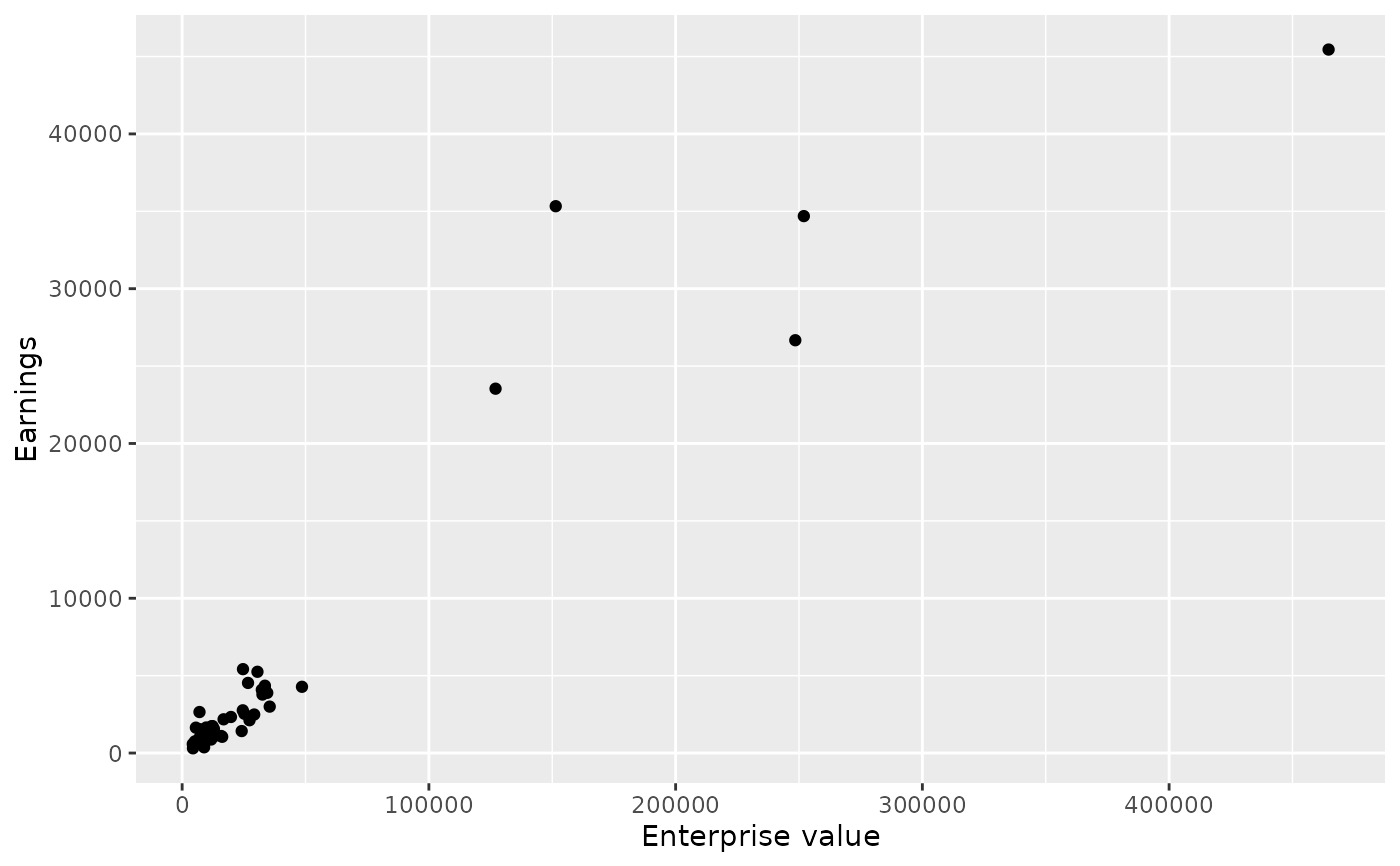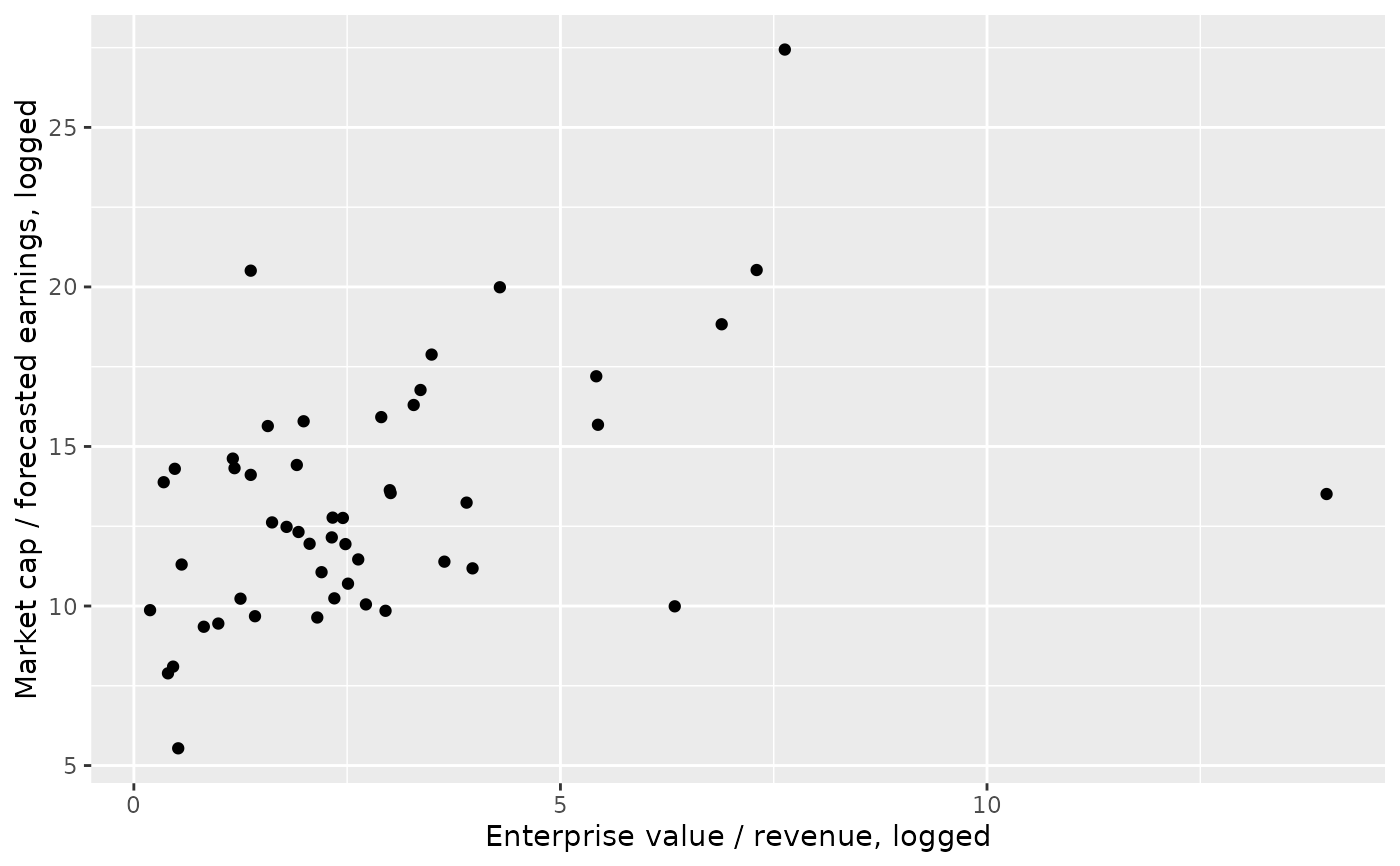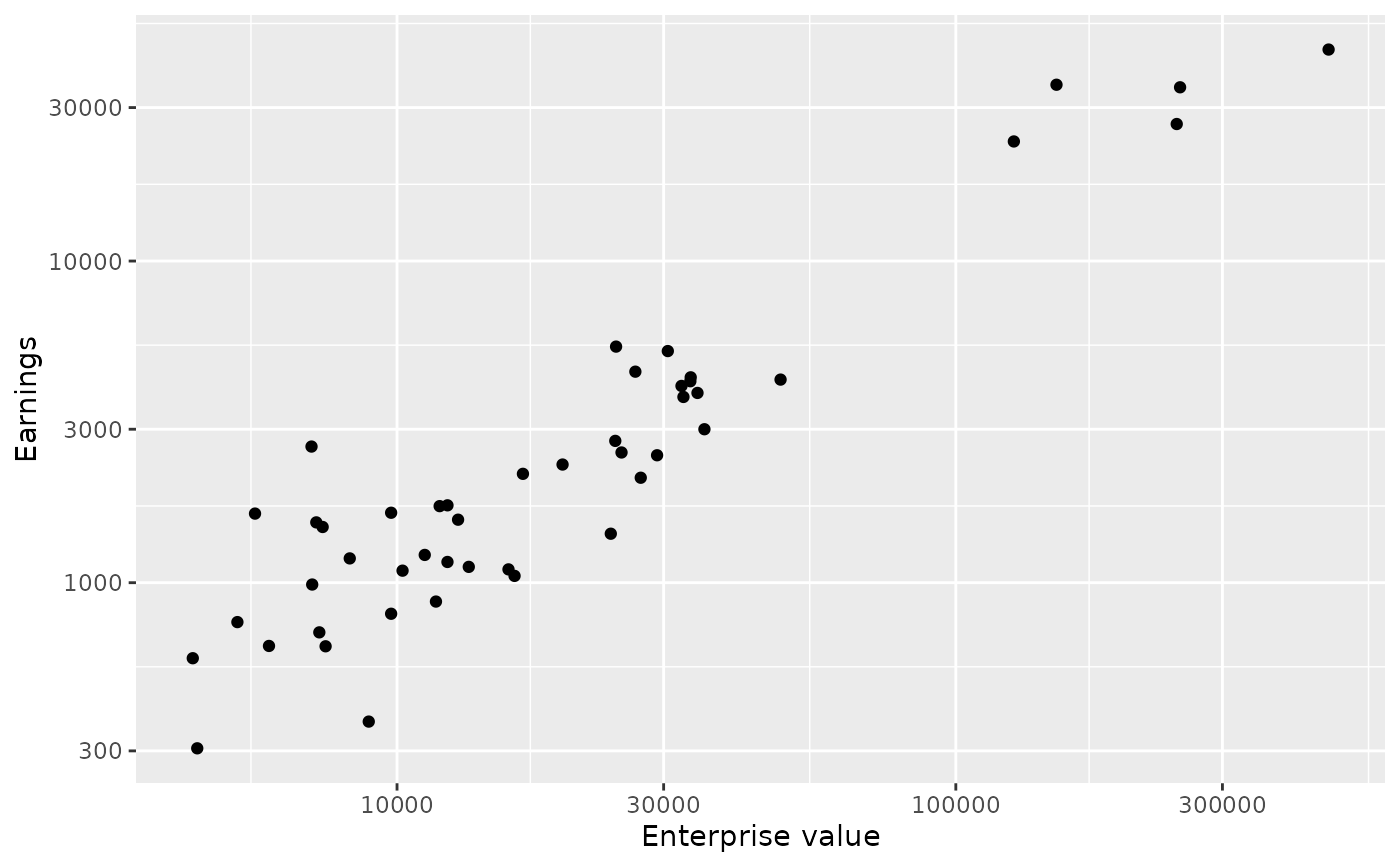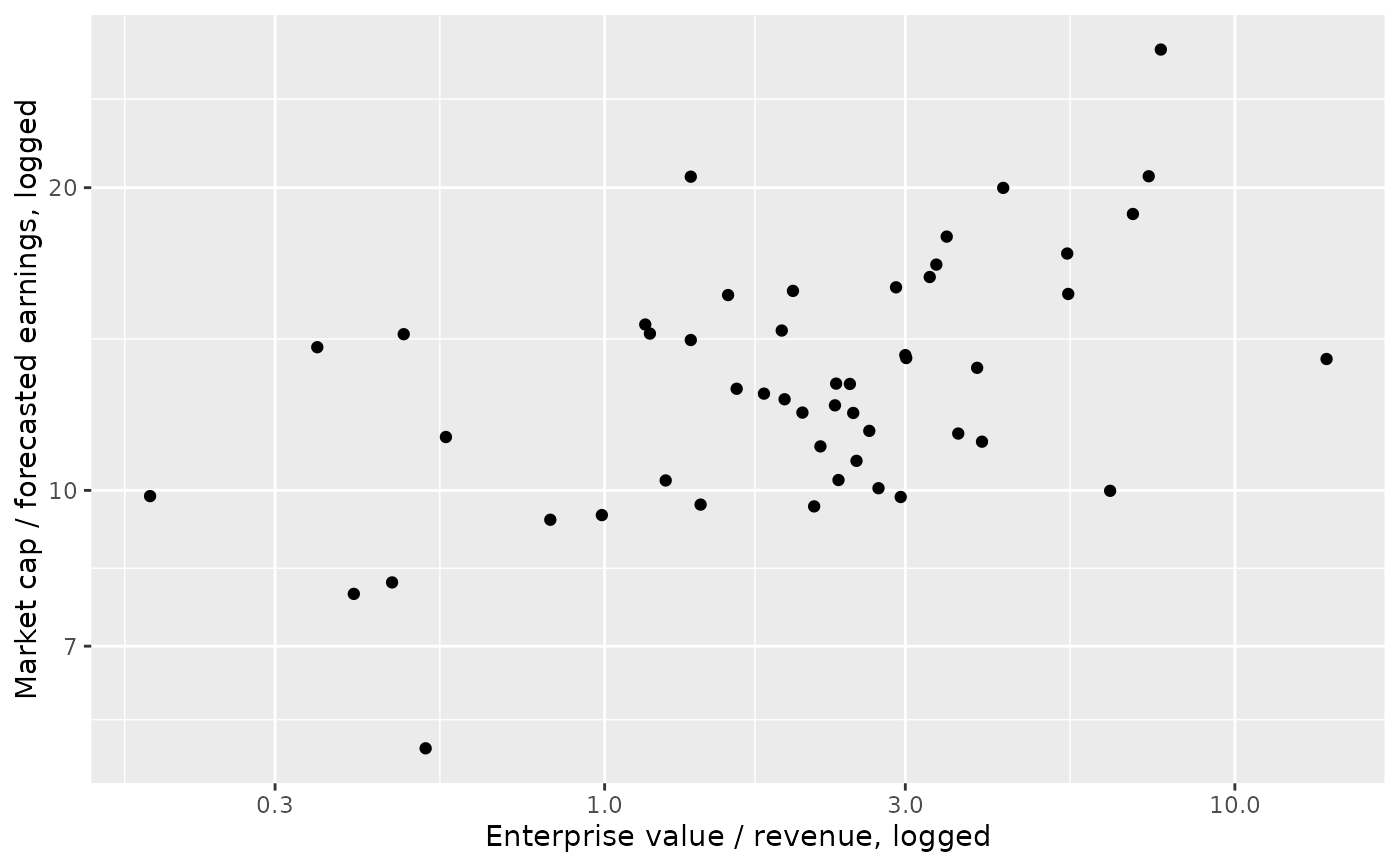Fifty companies were randomly sampled from the 500 companies in the S&P 500, and their financial information was collected on March 8, 2012.

## Usage

sp500

## Format

A data frame with 50 observations on the following 12 variables.

market_cap

Total value of all company shares, in millions of dollars.

stock

The name of the stock (e.g. AAPL for Apple).

ent_value

Enterprise value, which is an alternative to market cap that also accounts for things like cash and debt, in millions of dollars.

trail_pe

The market cap divided by the earnings (profits) over the last year.

forward_pe

The market cap divided by the forecasted earnings (profits) over the next year.

ev_over_rev

Enterprise value divided by the company's revenue.

profit_margin

Percent of earnings that are profits.

revenue

Revenue, in millions of dollars.

growth

Quartly revenue growth (year over year), in millions of dollars.

earn_before

Earnings before interest, taxes, depreciation, and amortization, in millions of dollars.

cash

Total cash, in millions of dollars.

debt

Total debt, in millions of dollars.

## Source

Yahoo! Finance, retrieved 2012-03-08.

## Examples


library(ggplot2)

ggplot(sp500, aes(x = ent_value, y = earn_before)) +
geom_point() +
labs(x = "Enterprise value", y = "Earnings")
#> Warning: Removed 3 rows containing missing values (geom_point).ggplot(sp500, aes(x = ev_over_rev, y = forward_pe)) +
geom_point() +
labs(
x = "Enterprise value / revenue, logged",
y = "Market cap / forecasted earnings, logged"
)ggplot(sp500, aes(x = ent_value, y = earn_before)) +
geom_point() +
scale_x_log10() +
scale_y_log10() +
labs(x = "Enterprise value", y = "Earnings")
#> Warning: Removed 3 rows containing missing values (geom_point).ggplot(sp500, aes(x = ev_over_rev, y = forward_pe)) +
geom_point() +
scale_x_log10() +
scale_y_log10() +
labs(
x = "Enterprise value / revenue, logged",
y = "Market cap / forecasted earnings, logged"
)